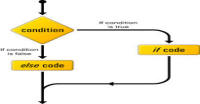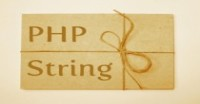# Operators in Ruby

There are a number of operators in ruby. Operators are those basic elements which do some operations on data like addition, subtraction, etc.

### Arithmetic Operators

These are mathematics operators.

 Operators Description Example + addition 4 + 5 = 9 - subtraction 12 - 34 = -22 * Multiplication 2*3 = 6 / Division, if both number are integers then integer is returned 3/2=1 but 3.0/2 = 1.5 % modulus, return reminder after division 5/2 = 1, 15/4 = 3 ** power 2**3 = 8 (2*2*2 = 8)

### Comparison Operators

These operators compare condition and return true or false.

 Operators Description Example == return true if both are equal otherwise false 2==2 is true != return true if both sides are not equal 2!=2 is false > return true if left side is greater 4>2 is true < return true if right side is greater 2<4 is true >= return true if right side is grater or equal 4>=4,5>=4, both true <= return true if left side is grater or equal 4<=4,3<=4, both true <=> return 0 if both equal, 1 if left greater and -1 if right greater 4<=>3 returns 1 === return true if right is in the range of left (1...20) === 9 returns true.

### Assignment Operators:

These operators assign the value to any variable after doing operation.

 Operator Description Example = assign value a = 12 += add right side to left side a+=b same as a = a+b -= subtract right side from left side a-=b same as a = a-b *= multiply right side to left side a*=b same as a = a*b /= divide right side by left side a/=b same as a = a/b %= divide right side by left side and assign reminder to right side a%=b same as a = a%b **= raise left side index as right side a**=b same as a = a**b

### Parallel Assignment:

In ruby, we can assign values to multiple variables in same line.

```# variables as well as values are saperated by comma.
name, age = 'harish',20
print "name is #{name} and age is #{age}"
```

Output:

```name is harish and age is 20
```

### Bitwise Operators:

If you are aware of binary numbers then ruby also provides bitwise operators. Lets take an example a = 23 (in binary, a =10111) and b = 43 (in binary, b = 101011). a&b; =3 (in binary, 000011). '&' is Binary AND operator. All operators are similar.

 Operators Description Example & Binary AND operator a&b; = 3 | Binary OR operator a|b = 63 ^ Binary XOR operator a^b = 60 ~ Binary AND operator ~23 = -24 >> Right shift operator 23>>2 = 95 << Left shift operator 23<<2 = 5

### Logical Operators:

These operators are also compare values and return true or false.

 Operators Description and both sides are true or at least one side is true && both sides are true || at least one side is true ! reverse the logical state, true becomes false not same as !

#### Click on banner below to Learn: PHP with Laravel for beginners - Become a Master in LaravelHarish, a technical core team member at www.lyflink.com with five year experience in full stack web and mobile development, spends most of his time on coding, reading, analysing and curiously following businesses environments. He is a non-graduate alumni from IIT Roorkee, Computer Science and frequently writes on both technical and business topics.

### Related ArticlesPHP stands for PHP: Hypertext Preprocessor. PHP is known as a server side scripting and interpreter language for web ...
##### Introduction to PHPIn this tutorial, we will study about conditional statements (if else statements and switch statements). These statem...
##### Conditional statements in phpIn this tutorial, we will study about strings and operations upon strings. Due to long length of this section, I have...

### The Complete Web Developer Course - Build 25 Websites